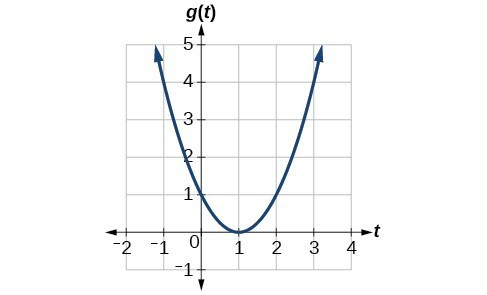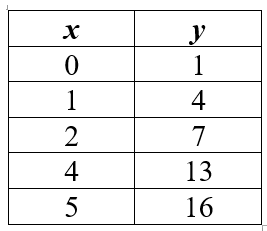Vocabulary
Rate of Change
Review
Intercepts
Linear or Nonlinear?
100

What do we call the order in which we simplify an expression?

What is order of operations(PEMDAS)?

100

What is the rate of change (slope) between (2,7) and (1,3)?

What is 4?

100

The solution to:  -2x - 13 = 7.

What is -5?

100
The y-intercept of y=5x+6

y=6 or (0,6)

100

The equation y=2x

what is linear?

200

This is the rise over the run.

What is the slope or rate of change?

200

What is the rate of change (slope) between (2,7) and (0,4)?

What is 3/2?

200

The solution to:  3x - 7 = 11

What is 6?

200

On a graph where can you find the x-intercept?

On the x-axis. Where the line crosses the x-axis.

200

This graph...What is nonlinear?

300

This is the point where the graph crosses the y-axis.

What is the y-intercept or initial value?

300

What is the rate of change (slope) in the table?What is 3?

300

A negative number times a negative number is always ____________.

What is positive?

300

The x-intercept of 2x=5y-10

x=-5 or (-5,0)

300

This table...What is nonlinear?

400

Define function.

What is a rule that defines a dependent relationship that pairs exactly one output for each input?

400

What is the rate of change (slope) in the graph?What is 2?

400

What is the test I can use to see if a graph is a function?

What is vertical line test?

400

The y-intercept of 2x-5y=-10

y=2 or (0,2)

400

This equation:

y= x2 + 15

What is nonlinear?

500

What is the input (x or y)?

What is the output (x or y)?

What is input x, output y?

500

What is the rate of change (slope)?What is 15?

500

What is 4 to the 3rd power?

What is 64?

500

The x-intercept of 2x-y=6

x=3 or (3,0)

500

This graph:What is linear?

Click to zoom